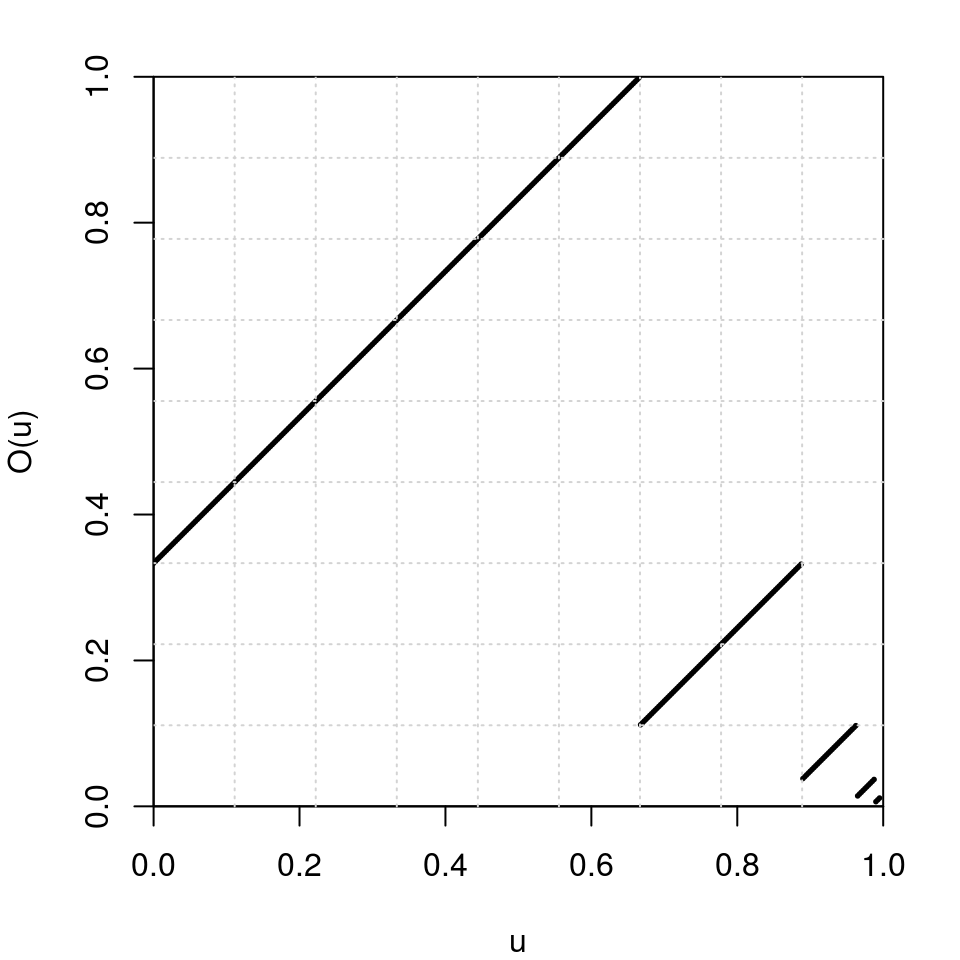1. The expansions package: integer and float expansions, and odometers

2016-08-13
Source

I released the expansions package for R. To install it, follow the instructions given on my repo.

Expansions of integer and real numbers

Conversion of decimal integers to integer base

This is the role of the intAtBase function:

intAtBase(14, base=3)
##  2 1 1
2*1 + 1*3 + 1*3^2
##  14

The intAtBasePower function returns the expansion of the integer $m^p$ where $m$ and $p$ are supplied by the user:

intAtBasePower(2, 5, base=3) == intAtBase(2^5, base=3)
##  TRUE TRUE TRUE TRUE

The advantage is the possibility to convert huge numbers $m^p$:

intAtBase(2^100, base=3)
## Error in intAtBase(2^100, base = 3): n is too big
intAtBasePower(2, 100, base=3)
##   1 2 2 0 1 1 1 1 2 2 0 2 2 0 1 1 0 0 2 1 0 2 0 2 1 0 0 2 2 1 0 1 1 0 0
##  0 0 1 1 1 2 2 1 0 0 0 0 2 2 2 1 2 0 2 1 0 1 0 2 2 2 0 0 1

The intAtBaseFib function returns the expansion of a Fibonacci number given by its index:

intAtBaseFib(6, base=3) == intAtBase(8, base=3)
##  TRUE TRUE
intAtBaseFib(100, base=3)
##   0 1 2 0 1 2 2 0 0 2 2 0 0 1 0 2 0 2 0 0 1 1 1 1 2 1 2 2 2 0 1 2 0 0 0
##  0 2 0 1 0 2 0 0 1

Cantor expansions, or “ary” expansions

The intToAry function performs the Cantor expansion of an integer. I explained the Cantor expansion in a previous article.

# (3,4,7)-expansion of 77:
intToAry(77, c(3,4,7))
##  2 1 6
2*1 + 1*3 + 6*(3*4)
##  77
# Cartesian product {0,1}x{0,1}:
sapply(0:3, function(x) intToAry(x, sizes=c(2,2)))
##      [,1] [,2] [,3] [,4]
## [1,]    0    1    0    1
## [2,]    0    0    1    1

It is implemented with Rcpp (source code), and it is fast.

Float expansions

The floatExpand01 function returns the expansion of a real number between $0$ and $1$ to its expansion in a given integer base. For example:

floatExpand01(0.625, base=2)
##  1 0 1

It means that $0.625 = 1\times \frac{1}{2} + 0 \times \frac{1}{2^2} + 1 \times \frac{1}{2^2}$.

The floatExpand function returns a list representing the expansion of a positive number in scientific notation: $x = 0.d_1d_2\ldots d_n \times \text{base}^e.$ The digits $d_1$, $\ldots$, $d_n$ are given in the first component of the list and the exponent $e$ is given in the second one. For example:

floatExpand(1.125, base=2)
## $digits ##  1 0 0 1 ## ##$exponent
##  1
(1*1/2 + 0*1/2^2 + 0*1/2^3 + 1*1/2^4) * 2^1
##  1.125

The odometer is the action $x \mapsto x+1$ on the group of $b$-adic integers. In other words, $x$ is the expansion in an integer base $b$ of a real number between $0$ and $1$, and the odometer maps $x$ to $x + (1, 0, 0, \ldots)$ where “$+$” is the addition modulo $b$ with carry to the right.

odometer(c(1,0,1), base=2)
##  0 1 1

By transporting the $3$-adic integers to $[0,1)$ with the float expansion in base $3$, let’s have a look at the graph of the $3$-adic odometer:

ternary2num <- function(t) sum(t/3^seq_along(t))
num2ternary <- function(u) floatExpand01(u, base=3)
par(mar=c(4,4,2,2))
u <- seq(0, 0.995, by=0.0025)
Ou <- sapply(u, function(u) ternary2num(odometer(num2ternary(u), base=3)))
plot(u, Ou, xlab="u", ylab="O(u)", xlim=c(0,1), ylim=c(0,1),
pch=19, cex=.25, pty="s", xaxs="i", yaxs="i")
grid(nx=9)The expansions package also provides the function sumadic to perform the sum of two adic integers: